﻿ Application of Advection Diffusion Equation for Determination of Contaminants in Aqueous Solution: A Mathematical AnalysisPublications are Open
Access in this journal
Article Versions
Export Article
• Normal Style
• MLA Style
• APA Style
• Chicago Style
Research Article
Open Access Peer-reviewed

### Application of Advection Diffusion Equation for Determination of Contaminants in Aqueous Solution: A Mathematical Analysis

Muhammad Masudur Rahaman, Humaira Takia , Md. Kamrul Hasan, Md. Bellal Hossain, Shamim Mia, Khokon Hossen
Applied Mathematics and Physics. 2022, 10(1), 24-31. DOI: 10.12691/amp-10-1-2
Received February 20, 2022; Revised March 22, 2022; Accepted March 29, 2022

### Abstract

Water pollution is one of the leading environmental issues faced especially at developing countries all over the World. However, the study of pollution movement is a necessary basis for solving water quality problems. The mathematical model for soluble and insoluble water pollutants has been formulated in light of partial differential equations. This research proposes the motion of flowing pollution by using a mathematical model in one-dimensional advection diffusion equation. Therefore, the study explored finite difference method for the numerical solutions of advection diffusion equation (ADE). Moreover, the study considered the ADE as an initial boundary value problem (IBVP) for the estimation of water pollution. By implementing a finite difference scheme for the IBVP, we examined as well as analyzed the extent of water pollution at different times and different points in an 1-dimensional spatial domain. We estimated relative error of the schemes in comparison with an exact solution of ADE and the numerical features of the rate of convergence are presented graphically. Finally, we compared our numerical solution of the ADE with solution obtained by finite element method. Numerical analysis indicates that water treatment is an essential tool for obtaining quality water for human consumption.

### 1. Introduction

Water is an important component of the earth. It covered two thirds of the earth’s surface. Almost all earth’s population, especially human being depend on freshwater to survive. Now-a-days, people are not only demand for the quantity of the water but also the quality of the water. Therefore, water quality has become an issue of increasing by the years 1.

Water pollution can be defined in many ways. Surface waters and groundwater are the two types of water resources which affected by pollution. Surface water such as rivers, lakes and oceans are the most useful sources to the human being and wildlife habitats 2. Most of water pollutions are caused by human activities. As stated by the World Health Organization (WHO) and United Nations Environment Program (UNEP), some examples of the activities which can cause water pollution are chemical fertilizers and chemical release by smokestacks. Farmers fertilized the fields and the chemical from the fertilizer used are washed by rained moves to the surface water nearby. Pollution also happen when chemical released by the smokestacks enter the atmosphere and they fall back to the earth as rain and entering the rivers, ocean or lake 1.

3 Stated that there are two techniques which can be applied to water transport problem, which are experimental and numerical modeling. Numerical modeling is considered to be more portable than experimental model. This is because, the parameters can be simply adjusted which make it easier to handle in industrial side. There are many numerical methods to solve the mathematical equations in order to deal with the water pollution problem. The mathematical models introduced can contribute to save the cost of human effort and material for a large number of chemical experiments to some degree 4.

An appropriate numerical method can give an accurate information on the concentration of pollution at different times and at different location efficiently and quickly 15. So, we have described advection diffusion equation as Mathematical Model for the estimation of the pollutants. Our intention is to investigate mathematical models and subsequent numerical methods for the estimation of the pollutants at different times and different points of water bodies caused by a source. This is the first time where we used these schemes FTCSCS (ECDS), FTBSCS (EUDS) and FTFSCS (EDDS) numerically to estimate pollutants in real life. Also in this study, FDM will be used for solving 1D ADE because of its ability to predict the concentration of pollutant.

The residual part of this paper is organized as: Section 2 includes the Modeling Equations; Section 3 shows Numerical Methods; Section 4 represents the results and discussion; and Section 5 summarizes the concluding remarks.

### 2. Modeling Equations

In this section we derived the advection diffusion equation using the method of superposition and demonstrated techniques to solve the resulting partial differential equation based on 16.

The derivation of the advection diffusion equation relies on the principle of superposition; advection and diffusion can be added together if they are linearly independent. Diffusion is a random process due to molecular motion. According to diffusion, each molecule in time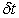will move.

• Figure 1. Schematic of a control volume with cross flow

Either one step to the left or one step to the right (i.e.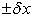). Due to advection, each molecule will also movein the cross-flow direction. These processes are clearly additive and independent; the presence of the cross flow does not bias the probability that the molecule will take a diffusive step to the right or the left; it just adds something to that step. The net movement of the molecule isand thus, the total flux in the x-direction(above shown in graph), including the advection transport and a Fickian diffusion term, must be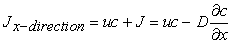Where,the correct form of the advection term.

We now use this flux law and the conservation of mass to derive the advection diffusion equation. Consider a cross flow velocity,as shown in Figure 1. From the conservation of mass, the net flux through the control volume is(1)

and for the x-direction, we have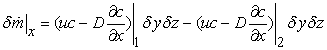We use linear Taylor series expansion to combine the two flux terms, giving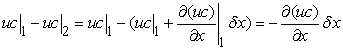And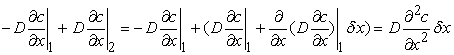Thus, for the x-directionThe y and z-directions are similar, but with v and w for the velocity components, giving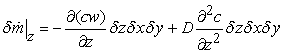Substituting these results into (2.5) and recalling that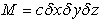We obtain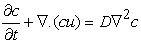or in Einsteinian notation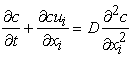In the one-dimensional case,and there are no concentration gradients in the y-direction or z-direction, leaving us with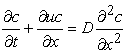Since u is constant, then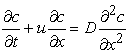(2)

It is well known Advection diffusion equation.

This mathematical model describing the transport and diffusion processes is the 1-dimensional advection-diffusion equation (ADE) with constant coefficients as well 8, 14, 17. Where,is the concentration at the point x at the time t, D is the diffusive constant in the x -direction, u is the fluid velocity.

Initial condition (I.C.) and boundary condition (B.C.), the following holds:

For I.C.:For B.C.: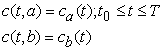### 3. Numerical Methods

Computer simulation techniques play an important role in the area of numerical solution method of the governing equations. We present the discretization of ADE as water pollution model as an IBVP. Let the solution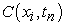be denoted by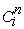and its approximate value bySimple approximations to the first derivative in the time direction can be obtained from forward difference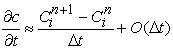Discretization ofis obtain from backward difference in spatial derivative.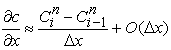The discretization ofis obtained by first order forward difference in spaceCentered difference discretization in spatial derivative: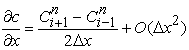The discretization ofis obtain from second order centered difference in space.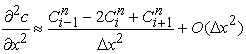For numerical solutions of ADE with initial and artificial or secondary boundary data, we study explicit centered difference scheme (FTCSCS), explicit upwind difference scheme (FTBSCS), explicit downwind difference scheme (FTFSCS). We implement the numerical schemes of advection diffusion equation by developing MATLAB code of computer programming for artificial initial and boundary data. We verify the stability conditions of the schemes and estimate the relative error of the numerical schemes for Advection diffusion equation. Finally we estimate concentration of water pollutant in river at different times and different points through advection-diffusion equation by FTCSCS.

### 4. Numerical Results and Discussion

We implement FTCSCS (ECDS), FTBSCS (EUDS) and FTFSCS (EDDS) finite difference schemes for solving advection diffusion reaction equation with artificial initial data, left boundary data and Neumann condition in right boundary data using . We have studied the qualitative behavior of water pollutant by choosing a river with length 25 m. The results are shown in the following figures.

• Figure 2. Concentration distribution at different time. Elapsed time 0.717672 sec
• Figure 3. Concentration distribution at different time. Elapsed time 0.714856 sec
• Figure 4. Concentration distribution at different time. Elapsed time 0.717252 sec
• Figure 5. Comparison among FTCSCS, FTBSCS and FTFSCS at different time

The Figure 1, Figure 2, Figure 3 and Figure 4 shows that the concentration of water pollutant distribution within the described domain. We have presented the numerical simulation results for pollutant transportation in river water by using finite difference scheme. We observed that pollutant concentration spreads out with respect to time and space. Therefore, the above realistic phenomenon is well described by our implementation. The analysis of numerical solution of ADE is important to capture spatial and temporal evolution of concentration of water pollutant in river and to ensure environmental sustainability and safety analysis. We can save our environment by research. The fish farm would have to take precaution to prevent contamination. The research findings contribute to realizing the sustainable development technology.

From the above table we stated that FTCSCS is stable for greater temporal step than FTBSCS and FTFSCS is stable for greater temporal step than FTCSCS. Finally FTCSCS is unstable later with increase of temporal size.

Our goal was to investigate the accuracy of numerical schemes for advection diffusion equation. We have discussed different types of finite difference scheme. In this section we have computed the relative error between analytic solution and different types of finite difference scheme to determine which scheme is best. We have computed the relative error in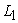-norm defined by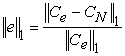for all time whereis the exact solution and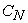is the Numerical solution computed by the explicit finite difference scheme.

• Figure 6. Numerical solution and analytic solution for ADE with
• Figure 7. Numerical solution and analytic solution for ADE

We have compared our numerical solution of the ADE with analytic solution in figure. We have noticed that the results obtained by the FTCSCS are in a good agreement with analytic solution.

• Figure 8. Comparison of relative errors among the schemes

The relative error for FTBSCS is below 0.04, the relative error for FTCSCS is below 0.002 and the relative error for FTFSCS is below 0.045. It is seen that the error by scheme FTCSCS is minimum. So, FTCSCS scheme is the best scheme. Finally, we implement the FTCSCS scheme for the estimation of pollutant transportation.

• Figure 9. Comparison of relative errors among the schemes with respect to discretization parameters size ∆t and ∆x

It is observed that relative error of the schemes is decreasing with respect to the smaller discretized parameters,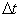and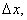which shows a good rate of convergence of the schemes. FTCSCS provides more accurate results than the FTBSCS and FTFSCS scheme with respect to discretization parameters sizeand. Numerical features of rate of convergence are performed and it is observed that the rate of convergence of FTCSCS is better than others scheme. Well understood qualitative behaviors are verified by which one can conclude that the FTCSCS scheme is compatible for the numerical estimation of the pollutant concentration by ADE.

The Table 2 displays the numerical solution of ADE with the values of diffusion coefficient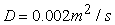and a flow in a long channel of water velocity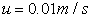for distance x = 42 meters and time t = 3000 seconds and compare the result with solution obtained by finite element method 17 using the same values of parameters in the same domain. In this case the initial boundary conditions are as followsand the constant boundary value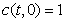.

We have compared our numerical solution of the ADE with solution obtained by finite element method 17. We have observed that the results obtained by the FTCSCS are good agreement with the results by finite elements method 17.

### 5. Conclusions

In this study, we have investigated finite difference method for the numerical solutions of advection diffusion equation (ADE). We have implemented FTBSCS, FTCSCS and FTFSCS schemes for the numerical solutions of ADRE as an IBVP. We have also given (FTCSCS) the explicit central difference scheme in space and forward difference method in time for the estimation of the generalized transport equation as advection diffusion equation. In error estimation of the numerical schemes for ADE, it is found that FTCSCS is more accurate than the other schemes. We have compared our numerical solution of the ADE with solution obtained by finite element method 17. It is observed that the results got by the FTCSCS are in a good agreement with the results by finite element method. Therefore, it is remarkable that the FTCSCS for ADE are suitable for the numerical estimation of water pollutant with realistic outcomes.

### Acknowledgements

The authors are thankful to Research and Training Centre (RTC) at Patuakhali Science and Technology University for the financial support.

### References

Published with license by Science and Education Publishing, Copyright © 2022 Muhammad Masudur Rahaman, Humaira Takia, Md. Kamrul Hasan, Md. Bellal Hossain, Shamim Mia and Khokon HossenThis work is licensed under a Creative Commons Attribution 4.0 International License. To view a copy of this license, visit http://creativecommons.org/licenses/by/4.0/